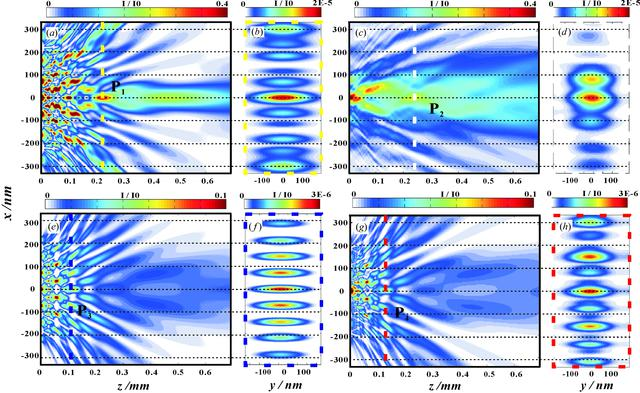disable zoom     view article Figure 8 Near-field distribution for (a), (b) the designed WGA, (c), (d) the measured WGA, (e), (f) the control structure WGM in theory and (g), (h) the measured WGM. (a) Design-WGA: one-dimensional FD simulation for the parameters of the designed WGA structure, showing the field in free space behind the exit plane of the WGA. (b) Design-WGA: the field distribution in the P1 xy plane, corresponding to the yellow dashed line in (a), calculated by full two-dimensional FD simulations. (c) Measured-WGA reconstructed by the loose support: one-dimensional free propagation by using the parabolic wave equation in 1 + 1 dimensions (x+z dimensions) (Fuhse & Salditt, 2005), starting from the reconstructed near-field pattern of the WGA [reconstruction data corresponding to Fig. 5(d)]. (d) Measured-WGA reconstructed by the loose support: the field distribution in the P3 xy plane, corresponding to the white dashed line in (c), calculated by the parabolic wave equation in 2 + 1 dimensions (xy+z dimensions). (e) Design-WGM: one-dimensional FD simulation for the parameters of the designed WGM structure, showing the field in free space behind the exit plane of the WGM. (f) Design-WGM: the field distribution in the P3 xy plane, corresponding to the dark-blue dashed line in (e), calculated by full two-dimensional FD simulations. (g) Measured-WGM: one-dimensional free propagation by using the parabolic wave equation in 1 + 1 dimensions, starting from the reconstructed near-field pattern of the WGM [reconstruction data corresponding to Fig. 7(c)]. (h) Measured-WGM: the field distribution in the P4 xy plane, corresponding to the red dashed line in (g), calculated by the parabolic wave equation in 2 + 1 dimensions.JOURNAL OFAPPLIEDCRYSTALLOGRAPHY
ISSN: 1600-5767
Volume 50| Part 3| June 2017| Pages 701-711